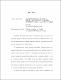## Algorithms for quantum simulation: design, analysis, implementation, and application2020
##### Authors
Su, Yuan
Simulating the Hamiltonian dynamics of quantum systems is one of the most promising applications of digital quantum computers. In this dissertation, we develop an understanding of quantum simulation algorithms concerning their design, analysis, implementation, and application. We implement three leading simulation algorithms, employing diverse techniques to tighten their error analyses and optimize circuit implementations. We produce concrete resource estimates for simulating a Heisenberg spin system, a problem arising in condensed matter physics that is otherwise difficult to solve on a classical computer. The resulting circuits are orders of magnitude smaller than those for the simplest classically-infeasible instances of factoring and quantum chemistry, suggesting the simulation of spin systems as a promising candidate for an early demonstration of practical quantum computation. We design new simulation algorithms by using classical randomness. We show that by simply randomizing how the terms in the Hamiltonian are ordered, one can prove stronger bounds for product formulas and thereby give more efficient quantum simulations. We also develop a classical sampler for time-dependent Hamiltonians, using which we give a simulation algorithm that substantially improves over previous approaches when the Hamiltonian varies significantly with time. We propose a general theory to analyzing product formulas, an approach to quantum simulation widely used in experimental demonstrations but whose error scaling was poorly understood. Our approach directly exploits the commutativity of Hamiltonian, overcoming the limitations of prior error analyses. We prove new speedups of product formulas for simulating many quantum systems, including simulations of nearest-neighbor lattice systems, second-quantized plane-wave electronic structure, $k$-local Hamiltonians, rapidly decaying power-law interactions, and clustered Hamiltonians, nearly matching or even outperforming the best previous results in quantum simulation. We accompany our analysis with numerical calculation, which suggests that the bounds also have nearly tight constant prefactors. We identify applications of quantum simulation to designing other quantum algorithms and improving quantum Monte Carlo methods. We develop an algorithmic framework quantum singular value transformation'' using techniques from quantum simulation and apply it to implement principal component regression. We also apply our new analysis of product formulas and obtain improved quantum Monte Carlo simulations of the transverse field Ising model and quantum ferromagnets.